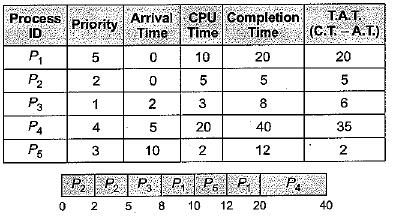Courses

# Introduction, Process,Threads And CPU Scheduling (Basic Level) - 1

## 10 Questions MCQ Test Question Bank for GATE Computer Science Engineering | Introduction, Process,Threads And CPU Scheduling (Basic Level) - 1

Description
This mock test of Introduction, Process,Threads And CPU Scheduling (Basic Level) - 1 for Computer Science Engineering (CSE) helps you for every Computer Science Engineering (CSE) entrance exam. This contains 10 Multiple Choice Questions for Computer Science Engineering (CSE) Introduction, Process,Threads And CPU Scheduling (Basic Level) - 1 (mcq) to study with solutions a complete question bank. The solved questions answers in this Introduction, Process,Threads And CPU Scheduling (Basic Level) - 1 quiz give you a good mix of easy questions and tough questions. Computer Science Engineering (CSE) students definitely take this Introduction, Process,Threads And CPU Scheduling (Basic Level) - 1 exercise for a better result in the exam. You can find other Introduction, Process,Threads And CPU Scheduling (Basic Level) - 1 extra questions, long questions & short questions for Computer Science Engineering (CSE) on EduRev as well by searching above.
QUESTION: 1

### Which of the following is an example of a SPOOLED device?

Solution:

Spool means simultaneous peri-pheral operations on line, a printer is a spooling device.

QUESTION: 2

### Concurrent processes are processes that

Solution:

Concurrent processes are processes that share the CPU and memory. They do overlap in time while execution. At a time CPU entertain only one process but it can switch to other without completing it as a whole.

QUESTION: 3

### Which of the following are real-time systems? 1. An on-line railway reservation system , 2. A process control system 3. Aircraft control system 4. Payroll processing system

Solution:

Real-time system are very fast and quick respondents systems. Response time of such systems is very low, to the tune of 10 ms or 100 ms or even less.

• Such systems are used where real-life scenario are being implemented like missile system, aircraft system extra.
• In process control system, the time quantum in reality is very less (to give a feel of multiprocessing so real time system is needed.
QUESTION: 4

In a time-sharing operating system, when the time slot given to a process is completed, the process goes from the RUNNING state to the

Solution: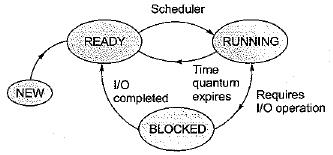In time-sharing operating system (example in Round-Robin), whenever the time slot given to a process expires, it goes back to READY state and if it requests for same I/O operation, then it goes to BLOCKED state.

QUESTION: 5

Which of the following are single-user operating system?

Solution:

MS-DOS (Microsoft Disk Operating System) is a single-user, single-tasking computer operating system that uses a command line interface. In spite of its very small size and relative simplicity, it is one of the most successful operating systems that has been developed to date.

QUESTION: 6

In a multiprogramming environment

Solution:

Multiprogramming environment means processor is executing multiple processes simultaneously by continuously switching between one-another. Therefore, multiple processes should reside in memory. However, processor can't executes more than one process at a time.

QUESTION: 7

Suppose that a process is in ‘BLOCKED’ state waiting for some I/O service. When the service is completed, it goes to the

Solution:

When process in blocked state waiting for some I/O services, whenever the services is completed it goes in the ready queue of the ready state.

QUESTION: 8

Consider a set of 5 processes whose arrival time, CPU time needed and the priority are given below: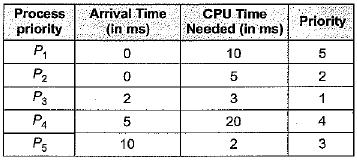Note: Smaller the number, higher the priority.

If the CPU scheduling policy is FCFS, the average waiting time will be

Solution:

Explanation : According to FCFS process solve are p1 p2 p3 p4 p5 so

for p1 waiting time =0 process time=10 then

for p2 waiting time = (process time of p1-arrival time of p2)=10-0=10 then

for p3 waiting time = (pr. time of (p1+p2)-arrival time of p3)=(10+5)-2=13 and

same for p4 waiting time=18-5=13

same for p5 waiting time=38-10=28

So total average waiting time = (0+10+13+13+28)/5

= 12.8

QUESTION: 9

Consider a set of 5 processes whose arrival time, CPU time needed and the priority are given below:Note: Smaller the number, higher the priority.

If the CPU scheduling policy is SJF, the average waiting time (without pre-emption) will be

Solution: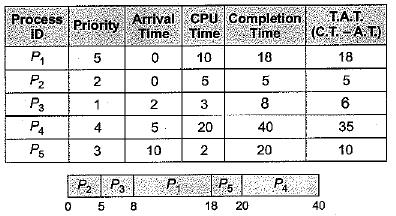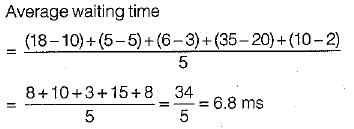QUESTION: 10

Consider a set of 5 processes whose arrival time, CPU time needed and the priority are given below:Note: Smaller the number, higher the priority.

If the CPU scheduling policy is SJF with preemption, the average waiting time will be

Solution: